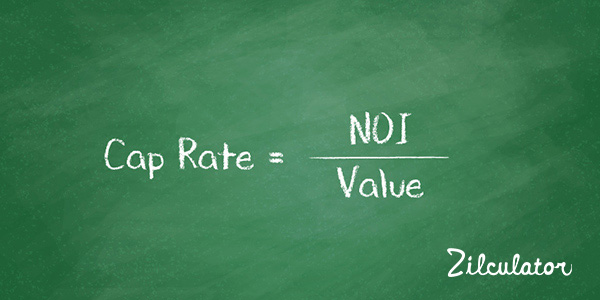# How to Calculate Cap Rate

## Formula & DefinitionCap rate (or Capitalization rate) is the rate at which you discount future income to determine its present value. In practice, you will typically use cap rate to express the relationship between a property's value and its net operating income (NOI) for the current or coming year.

You can use the cap rate formula to serve one of two most typical purposes:

1. Obviously, you can use it to calculate a property's cap rate. You will want to do so when you know its NOI and what is presumably its current market value - probably an After Repair Value or a seller's asking price. What you are really doing in this situation is finding out if the property exhibits a cap rate that is in line with other similar properties in the area if you purchase it at the asking price.
2. If you know what is an appropriate cap rate for this type of property in this area, then you can transpose the formula to calculate a reasonable estimate of value. In other words, forget about the seller's asking price; given the NOI and the prevailing cap rate, what should it be worth?

Zilculator helps real estate professionals calculate cap rate easily. Never use a spreadsheet again! Analyze your own property or create investment reports for your clients.

• Sales and Rental comps

## How to Calculate Cap Rate

1. Calculate Net Operating Income of the property (see a separate article How to Calculate NOI)
2. Determine current Value of the property, probably an After Repair Value or a seller's asking price.
3. Property's simple or market Cap Rate is the ratio between these two: